##### Algebra II For Dummies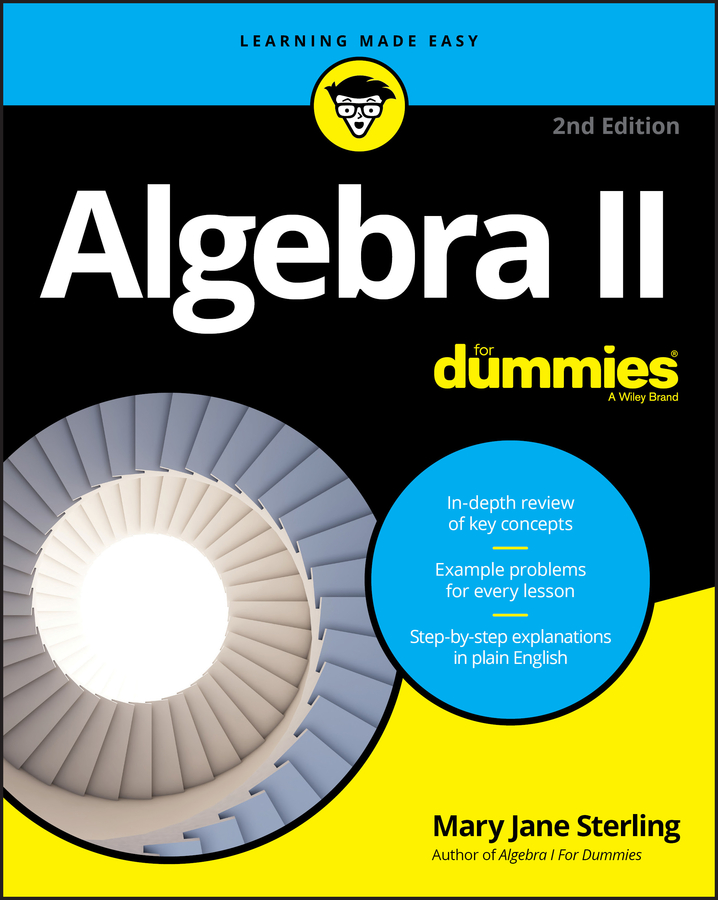You can compare number values more easily when you use exponents, because numbers — especially large ones — can be deceiving. To discover the real value of a large number, write it as a number between 1 and 10, times a power of 10. Writing a number as an exponential expression makes it easy to compare to other numbers — the number with the higher exponent is the larger number.

For example, compare two numbers: 943,260,000,000,000,000,000,000 and 8,720,000,000,000,000,000,000,000.

1. Write them as numbers between 1 and 10, times a power of ten.

943,260,000,000,000,000,000,000 = 9.4326 × 100,000,000,000,000,000,000,000

8,720,000,000,000,000,000,000,000 = 8.72 × 1,000,000,000,000,000,000,000,000

2. Write each power of ten as an exponential expression with the exponent indicating the number of zeros to use.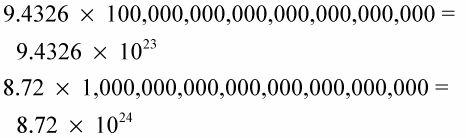3. Compare the numbers.

The number with the higher power of ten — the larger exponent — is the larger number.

In this case, the exponent is 24 for the expression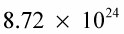and the exponent is 23 for the expression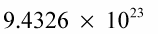Because 24 is greater than 23,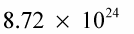is the larger number.

If the powers are the same, then compare the numbers that precede the multiplication sign. For example, if you compare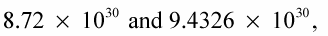you see that both powers are the same — the exponent for each expression is 30. Because exponents for both expressions are the same, you have to compare the numbers that precede the multiplication signs: 8.72 and 9.4326. Because the number 8.72 is smaller than 9.4326,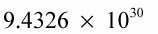is the larger number.